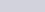ChemTalk

# Galvanic Cells & Voltaic Cells## Core Concepts

In this tutorial on galvanic cells, aka voltaic cells, you will learn the basics of redox reactions and how to apply this information to electrochemical cells. You will also learn how to determine what half-reactions occur at which electrode and use these standard half-reaction potentials to calculate cell potential.

There are two types of electrochemical cells – a voltaic cell, also called a galvanic cell, and an electrolytic cell. Voltaic cells produce electricity, while electrolytic cells use a power source to drive a reaction forward.

## Basics of Oxidation-Reduction Reactions

In an electrochemical process, electrons flow from one substance to another substance in what is known as an oxidation-reduction (redox) reaction. Redox reactions happen when a substance called an oxidizing agent oxidizes another substance by taking electrons and being reduced. In a redox reaction, a simple mnemonic to remember the direction of the transfer of electrons is “Oil Rig,” which stands for “Oxidation is losing, reduction is gaining.” This refers to the fact that in oxidation, a species loses electrons while in reduction, the species gains electrons.

In reactions that are not redox reactions, like acid-base reactions, the oxidation states of the elements do not change.

A simple example of an oxidation reaction is the reaction of iron (II) oxide with carbon monoxide as follows: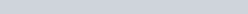In this reaction, iron is reduced from an oxidation state of +3 to 0, while carbon is oxidized from an oxidation state of +2 to +4. All redox reactions will have one species being oxidized and one species reduced.

Some elements, like vanadium, can even progress through multiple oxidation states as a redox reaction progresses.

## Reduction Potential

Potential, also known as voltage, is a very important concept in electrochemistry. It represents the energy in Joules transferred by one Coulomb of charge. Every chemical species, including atoms and ions, has a certain “willingness” to accept electrons. This is called the standard reduction potential, and it’s measured in volts (J/C). The reduction potential is different than electronegativity and electron affinity, but is related to both. The more positive the reduction potential, the more favored a reduction is.

Because the reduction half-reaction is the process by which an atom/ion accepts an electron, the favorability of that half-reaction can be represented by the reduction potential. Conversely, the oxidation half-reaction shows the opposite of an electron being accepted, so it will be represented by the negative of the reduction potential. Summing the oxidation and reduction half-reactions gives the overall redox reaction equation. In the same way, summing the reduction and oxidation potentials gives the potential for the overall reaction. The potential is static for a given half-reaction. Because the value never changes, tables of standard reduction potentials are an electrochemist’s best friend.

### Calculating the potential

The notation for voltage in this context is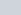(“e-naught”).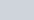denotes reduction potential and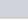denotes oxidation., or the overall potential for the reaction, is simply the sum ofand. Here’s an example putting this all together:

Reduction half-reaction: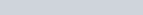From the table, this half-reaction has a potential of +1.36 volts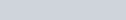.

Oxidation half-reaction: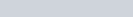From the table, the reduction of aluminum has a voltage of -1.66 volts. However, this is the OXIDATION of aluminum, NOT the reduction. Therefore, the opposite voltage is used, so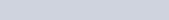.

Net redox reaction: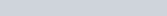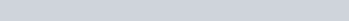#### But wait, the stoichiometry…

Some may be wondering “if the reduction half-reaction is multiplied by three for the net reaction, why isn’t the potential multiplied by three?” Reduction potential is one of the few places in chemistry that stoichiometry doesn’t apply. The reason why is simple: remember that voltage is a ratio. “How much energy is transferred per coulomb of charge?” While three times as many chlorine atoms must be reduced than aluminum, the stability imparted by that transformation (the “willingness”), is the same for each chlorine electron. Therefore, the sheer number of chlorine electrons does not affect the energy change seen by any given one.

## Electrochemical Cells

There are two types of electrochemical cells: galvanic and electrolytic.

The galvanic cell has a long history. Its name comes from Italian physicist Luigi Galvani (1737 – 1798), who observed that dissected frog leg muscles twitched when an electric current was applied to them. A galvanic cell uses the energy released from a spontaneous redox reaction to produce an electric current. Another common name for galvanic cells is voltaic cells, which is named after another Italian physicist, Alessandro Volta (1745 – 1827), who invented the galvanic (voltaic) cell.

On the other hand, an electrolytic cell uses an electric current to drive a normally non-spontaneous redox reaction. A typical example of an electrolytic cell is applying an electrical current to water to drive the decomposition of water into hydrogen and oxygen gases through the following reaction:

(1)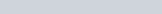The main similarities between galvanic and electrolytic cells are that they both use two electrodes, an anode and a cathode, and contain electrolyte solution. This electrolyte solution allows the transfer of ions in and out of solution while keeping the overall charge of the cell neutral.

The major difference between a galvanic and electrolytic cell is the direction of work. A galvanic cell turns a spontaneous chemical reaction into usable work, whereas an electrolytic cell uses work to drive a non-spontaneous reaction.Oxidation occurs at the anode while reduction occurs at the cathode. An easy mnemonic to remember this is “The red cat and an ox,” where “red cat” means reduction – cathode, and “an ox” means anode – oxidation.

## Galvanic Cells

A galvanic cell is a setup that facilitates redox reactions in a controlled and specific way that generates a current (flow of electrons). Voltaic cell, electrochemical cell, and battery are all different names for a galvanic cell. Below is a typical galvanic cell that will demonstrate all the important features.

Every galvanic cell MUST contain the following:

• An oxidation half-cell, where electrons will be lost
• A reduction half-cell, where electrons will be accepted
• A wire connecting the two cells, often containing a voltmeter to measure the potential of the cell
• A salt bridge or some other way of maintaining charge balance

Let’s go over each of these features specifically and why they are necessary.

### The oxidation half-cell

The oxidation half-cell is the place electrons originate. It consists of two main parts: the electrode and the solution. The electrode of an oxidation cell is called the anode. In this example the anode is zinc. As the reaction occurs, electrons leave the zinc metal in the anode, converting it to zinc ions. These ions then enter the solution, in this case zinc sulfate. The counterion doesn’t matter, as long as it’s soluble with the anode metal ion. The cation of the solution however should match the metal of the anode. During the reaction, electrons leave the anode and travel over the wire to the reduction half-cell, increasing the concentration of metal ions in the solution and eating away the anode.

### The wire

This is the simplest part of the setup. The wire allows electrons to travel between the two half-cells. Often it connects to a device called a voltmeter which measures the potential. Voltmeters are useful for monitoring the progress of the reaction, but not required. The conductor doesn’t HAVE to be a wire, but wires are the most common way of connecting the cells for these types of setups.

### The reduction half-cell

The reduction half-cell is the destination for the electrons liberated from the anode. The metal electrode in the reduction half-cell is called the cathode. As electrons travel down the cathode, they contact the ion solution. The cations in the solution are then reduced to metal and deposit on the surface of the cathode. In a simple galvanic cell, the cations match the cathode. In this example, copper is used for the cathode, and therefore the cations in the solution are copper. Again, the counterion doesn’t matter. As the reaction proceeds, the concentration of the cation goes down and the mass of the cathode increases.

### The salt bridge

As the reaction proceeds, electrons leave the anode and travel to the cathode. The positive charge from metal ions in the oxidation half-cell increases, and decreases in the cathode half-cell. This is an issue, because charge buildups make reactions unfavorable most of the time. The salt bridge removes this problem. Generally, simple spectator ion salts like KCl, NaNO3, et cetera make for good salt bridges. The salt bridge is saturated with these compounds and will balance out the charge in the two half-cells. In the example shown, the salt may contain potassium sulfate. As the reaction proceeds, potassium ions will enter the reduction half-cell to replace lost copper ions. Likewise, sulfate ions will enter the oxidation half-cell to balance out the zinc ions entering the solution. Without a salt bridge to mediate charge, galvanic cells do not work.

## Galvanic Cells Example Problem

A chemist sets up a galvanic cell to mediate the reaction below: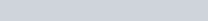They use sodium ions to mediate positive charge and nitrate as every counterion. Label each part of the cell with the name and chemical species where appropriate, and list the voltage associated with the reaction under standard conditions.

a: voltmeter

b: NaNO3 salt bridge

c: Cr anode

d: Cr(NO3)3 solution

e: Ag cathode

f: AgNO3 solution

Voltage: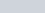## Determining Potential of Galvanic Cells

Consider the classic Cu-Zn galvanic cell (voltaic cell) pictured below

As you can see from the image, there are two electrodes. One of them is zinc, and the other one is copper. We saw from the example before that zinc is the anode and copper is the cathode. But how do we know? In order to determine which electrode is the anode and which is the cathode, the two half-reactions must be considered. A half-reaction is the net reaction that occurs with the species being oxidized or reduced and shows the flow of electrons. Take the two half-reactions in the Cu-Zn cell: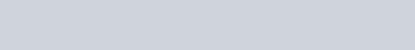When given two half-reactions like this, the species with a higher reduction potential will typically be reduced. Because of this, we can see that in the Cu-Zn cell, the copper will be reduced while the zinc will be oxidized. Next, we must flip the zinc half-reaction to show the oxidation reaction: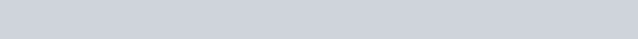Note that although we flipped the chemical equation, the standard reduction potential remains negative.

From the copper reduction half-reaction and the zinc oxidation half-reaction, we can create the overall net redox reaction by adding them together and making sure the electrons add up properly:We can then use the equation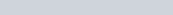to determine the overall potential of the cell. For our Cu-Zn cell, the potential is: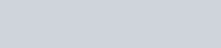Recall that reduction occurs at the cathode and oxidation occurs at the anode. You can read more about calculating cell potentials here.

## Cell Diagrams

Understanding a cell diagram is very simple. The concept of a cell diagram is to give a more convenient line notation that easily shows the phase boundaries, notated by a single vertical line, and the salt bridge, notated by a double vertical line.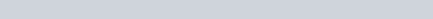Following this convention for our Cu-Zn cell would lead us to the following cell diagram: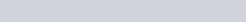## Galvanic Cells Practice Problems

Problem 1

In a galvanic cell, you have silver and aluminum, which obey the following redox reactions: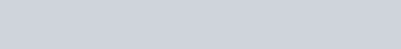For the galvanic cell to work, which metal is present at the anode and which is present at the cathode?

Problem 2

You have a galvanic cell with a magnesium anode and a chromium cathode.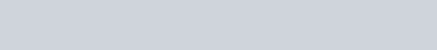Calculate the cell potential

## Galvanic Cells Practice Problems

1: Anode: Al; Cathode: Ag

2: